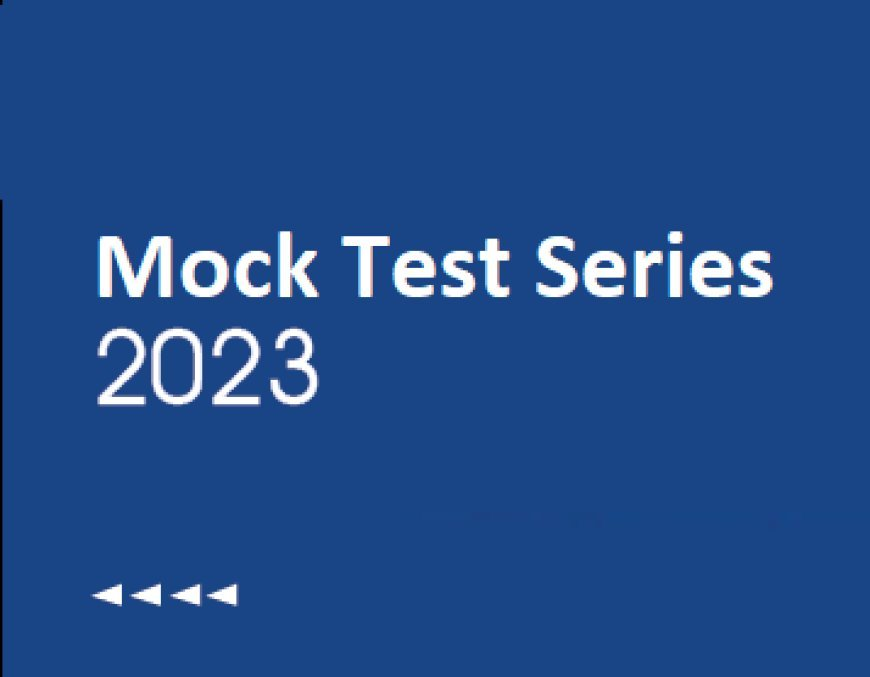# AAO Exam-CT 2: Quant (Number Series)

## AAO Exam-CT 2- Quant (Number Series)

0  1471. What should come in place of the question mark '?' in the following number series? 126, 147, 168, ?, 210, 231

• 178
• 196
• 190
• 175
• 189

Calculation

The series follows following pattern

147 - 126 = 21

168 - 147 = 21

189 - 168 = 21

210 - 189 = 21

231 - 210 = 21 ∴ The value of ? is 189

2. What should come in place of the question mark '?' in the following number series? 17, 90, 273, 274, -275, 822, ?

• 3125
• -3125
• 4115
• -4115
• -4225

Calculation

The series follows following pattern

17 × 5 + 5 = 90

90 × 3 + 3 = 273

273 × 1 + 1 = 274

274 × (-1) - 1 = -275

-275 × (-3) - 3 = 822

822 × (-5) - 5 = -4115 ∴ The value of ? is -4115

3. What should come in place of the question mark '?' in the following number series? 3, -2, 6, -15, ?, -315

• 62
• 64
• 63
• 65
• 60

Calculation

The series follows following pattern

⇒ 3 × (-1) + 1 = -2

⇒ -2 × (-2) + 2 = 6

⇒ 6 × (-3) + 3 = -15

⇒ -15 × (-4) + 4 = 64

⇒ 64 × (-5) + 5 = -315

The value of ? is 64

4. What should come in place of the question mark '?' in the following number series? 3, 7, 15, ?, 43, 63

• 32
• 25
• 27
• 36
• 21

Calculation

The series follows following pattern

3 + (1 × 4) = 7

7 + (2 × 4) = 15

15 + (3 × 4) = 27

27 + (4 × 4) = 43

43 + (5 × 4) = 63

The value of ? is 27

5. What should come in place of the question mark ‘?’ in the following number series?

17, 19, 40, 122, ?, 2452

• 500
• 490
• 530
• 570
• None of these

Calculation:

The series follows the following pattern

17 × 1 + 2 = 19

19 × 2 + 2 = 40

40 × 3 + 2 = 122

122 × 4 + 2 = 490

490 × 5 + 2 = 2452

The missing number is 490.

6. In each of the following number series, the wrong number is given, find out that number.

3, 7, 22, 70, 206, 537

• 3
• 7
• 206
• 70
• 537

The series follows the following pattern

3

⇒ 3 + 13 + 3 = 7

⇒ 7 + 23 + 7 = 22

⇒ 22 + 33 + 22 = 71

⇒ 71 + 43 + 71 = 206

⇒ 206 + 53 + 206 = 537

the wrong number in the series is 70

7. In each of the following number series, the wrong number is given, find out that number.

22, 21, 41, 128, 513, 2564

• 128
• 513
• 2564
• 21
• 41

The series follows the following pattern:

22 × 1 - 1 = 21

21 × 2 + 1 = 43

43 × 3 - 1 = 128

128 × 4 + 1 = 513

513 × 5 - 1 = 2564

∴ The wrong term in the series is 41.

8. In each of the following number series, the wrong number is given, find out that number.

1440, 240, 48, 16, 4, 2

• 240
• 2
• 4
• 16
• 48

The series follows the following pattern:

1440 ÷ 6 = 240

240 ÷ 5 = 48

48 ÷ 4 = 12

12 ÷ 3 = 4

4 ÷ 2 = 2

∴ The wrong term in the series is 16.

9. In each of the following number series, the wrong number is given, find out that number.

35, 50, 63, 80, 99, 122

• 63
• 80
• 122
• 99
• 50

The series follows the following pattern:

62 – 1 = 35

72 + 1 = 50

8– 1 = 63

92 + 1 = 82

102 – 1 = 99

112 + 1 = 122

∴ The wrong term in the series is 80.

10. In each of the following number series, a wrong number is given. Find out that number.

24, 47, 45, 91, 90, 179

• 91
• 47
• 179
• 90
• 45

The series follows the following pattern:

24 × 2 - 1 = 47

47 × 1 - 1 = 46

46 × 2 - 1 = 91

91 × 1 - 1 = 90

90 × 2 - 1 = 179

∴ The wrong term in the series is 45.

### AAO Exam-Quantitative Aptitude (Mock Test)